# Numerical Solution of Natural Convection on a Vertical Stretching Surface with Suction and Blowing

Numerical Solution of Natural Convection on a Vertical Stretching Surface with Suction and Blowing

Shankar Goud BejawadaYanala Dharmendar Reddy Kanti Sandeep Kumar Epuri Ranjith Kumar

Department of Mathematics, JNTUH College of Engineering Hyderabad, Kukatpally, Telangana 500085, India

Department of Mathematics, Anurag University, Hyderabad, Telangana 500088, India

Department of Mathematics, Hyderabad Institute of Technology and Management, Hyderabad, Telangana 501401, India

Department of Mathematics, Kakatiya Institute of Technology and Science, Warangal 506371, Telangana, India

Corresponding Author Email:
bsgoud.mtech@gmail.com
Page:
1469-1474
|
DOI:
https://doi.org/10.18280/ijht.390508
23 March 2020
|
Accepted:
23 August 2021
|
Published:
31 October 2021
| Citation

OPEN ACCESS

Abstract:

In this paper, the natural convective heat transfer from a stretching sheet oriented vertically involving surface mass transfer is of primary focus. A similarity solution in three dimensions is described for energy and momentum. The transformed equations are answered by using MATLAB in-built numerical programmer solver bvp4c. For a range of Prandtl numbers and surface mass transfer rates, friction factor and Nusselt numbers are tabulated. The heat transfer mechanism is observed to influence surface mass transfer. Heat transfer rate increases and thermal boundary layer thickness decreases with an increase of Prandtl values. In addition, the current results are compared with the previously published results and initiate to be a successful agreement.In this paper, the natural convective heat transfer from a stretching sheet oriented vertically involving surface mass transfer is of primary focus. A similarity solution in three dimensions is described for energy and momentum. The transformed equations are answered by using MATLAB in-built numerical programmer solver bvp4c. For a range of Prandtl numbers and surface mass transfer rates, friction factor and Nusselt numbers are tabulated. The heat transfer mechanism is observed to influence surface mass transfer. Heat transfer rate increases and thermal boundary layer thickness decreases with an increase of Prandtl values. In addition, the current results are compared with the previously published results and initiate to be a successful agreement.

Keywords:

stretching sheet, heat transfer, natural convection, steady flow, friction factor

1. Introduction

Heat transfer by natural convection frequently occurs in many physical problems and engineering applications, Viscous fluid flow studies are conducted in many practical applications with heat transmission due to stretching surface areas in production, where manufacturing process of plastic and rubber sheet through the non-functional product. Other notable applications of the study are spinning of fibers, Extrusion processes, continuous coating, and glass blowing. This was noted by some of the researchers viz., Surma Devi et al.  analyzed on a stretching sheet of an unsteady, 3-D boundary-layer flow. Heat and mass transfer on a stretching sheet with suction or blowing was examined by Gupta and Gupta , Chen and Char , and Vajravelu . Bhattacharyya  shows the heat source/sink impacts of MHD flow and the transfer of heat over a shrink layer in the existence of mass suction. Jat and Chaudhary  investigated a stretching sheet with heat transfer on MHD flow. Fathizadeh et al.  have considered MHD viscous flow and heat transfer over a stretching sheet with an effective modification of the homotopy perturbation. Heat transfer over a steady stretching surface with suction was analyzed by Siri et al. . Daskalakis  then studied free convection effects in the boundary layer along a vertically stretching flat surface. Similarity answer on MHD boundary layer across stretching surface considering thermal flux was studied by Ferdows et al. . Goud and Shekar  have studied the effects on unsteady MHD flow past a parabolic started vertical plate with mass diffusion and viscous dissipation with variable temperature by the technique of finite element process. Wang  presented free convection on a perpendicular stretching surface. Bestman  investigated the natural convection boundary layer in a porous medium with mass transfer and the effect of suction. Acharya et al.  have studied the suction and blowing effect on an acceleration surface with heat and mass transfer. An unsteady MHD flow past an impulsive inclined oscillating plate with the impact of viscous dissipation and diffusion thermo in the presence of variable temperature was deliberated by Goud and Rajahekar . Ali  investigated the influence of suction/injection on the stretched surface power-law thermal boundary. Free convection boundary layer over a vertical porous flat plate with internal thermal generation in a permeable medium analyzed by Postelnicu et al. . Shateyi  presented the buoyancy impacts and thermal radiation on heat & mass transfer through a semi-infinite stretching surface with suction and blowing. Chemically reactive solutions flow across the MHD boundary layer over a permissible stretching sheet with suction or blow have been explored by Bhattacharyya and Layek . Goud and Rajashekar  presented an unsteady MHD boundary flow on the semi-infinite vertical with thermal source and suction effects via porous medium outcomes obtained by implementing the finite element method. Some researchers have studied the suction/ injection and other aspects also ref. [21-29].

The current work is about an incompressible, natural convective heat transfer 3-D steady flow past on a stretching sheet involving blowing and suction. Using Boussinesq assumptions, a 3-D similarity result in the Navier-Stokes equations is reported furthermore, the current results are consistent with previously reported outcomes and seem to be a good contract in a defining context Gorla and Sidawi . The governing equations are eased to a couple of non-linear ODEs that are explained using a MATLAB solver.

2. Mathematical Formulation

In this study, consider an incompressible, steady fluid flowing with a velocity u=bx past a plane stretching perpendicular to the x-direction. Also, consider that the y-axis of the sheet makes an angle α with the horizontal and z-axis of the sheet is normal to the sheet. Components of velocity are assumed by ‘u’ in the x-direction, ‘v’ in the y-direction, and ‘w’ in the z-direction. Figure 1 can be used as a reference for the flow model and coordinate system. Let us neglect effects along the edges and hence all the variables along the y-direction are independent. Therefore, the Boussinesq approximation governing equations are:

## 1.png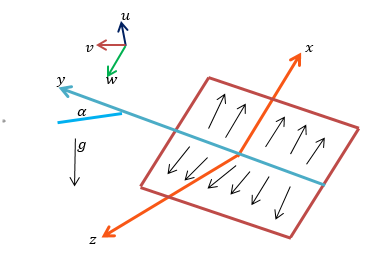Figure 1. Scheme of a coordinate system and flow model

Momentum:

$\frac{\partial u}{\partial x}+\frac{\partial w}{\partial z}=0$                    (1)

$u\frac{\partial u}{\partial x}+w\frac{\partial w}{\partial z}=g\beta \left( T-{{T}_{\infty }} \right)\cos \alpha +v\frac{{{\partial }^{2}}u}{\partial {{x}^{2}}}$                (2)

$w\frac{\partial \upsilon }{\partial z}=g\beta \left( T-{{T}_{\infty }} \right)\sin \alpha +v\frac{{{\partial }^{2}}\upsilon }{\partial {{z}^{2}}}$                 (3)

$w\frac{\partial T}{\partial z}=-\frac{1}{\rho }\frac{\partial p}{\partial z}+v\frac{{{\partial }^{2}}w}{\partial {{z}^{2}}}$                 (4)

Energy:

$w\frac{\partial T}{\partial z}=\frac{v}{\Pr }\frac{{{\partial }^{2}}T}{\partial {{z}^{2}}}$                 (5)

The following are the boundary conditions to be applied:

$\left.\left.\begin{array}{c}u=b x, \\ v=0, \\ w=W, \\ T=T_{w}\end{array}\right\}_{z=0}; \,\, \begin{array}{c}u=0 \\ v=0 \\ \partial w / \partial z=0 \\ T=T_{\infty}:\end{array}\right\}_{z \rightarrow \infty}$

3. Coordinate Transform

When similarity solutions are used, the number of free variables is summarized by one or more. This is the most probably used method in fluid mechanics to obtain exact solutions. Ames  describes the methods for equations of physical interest in generating similarity transformations. In the field of limiting solutions, asymptotic solutions are used as similarity solutions. Physical insight into these specifics of complex flows of fluid may be obtained by using similarity solutions for complex fluid flows. Most of the characteristics exhibited by these solutions describe the actual problem for the physical, dynamic, and thermal parameters.

$u=b x f^{\prime}(\eta)+A \cos \alpha \cdot \psi(\eta), \quad v=A \sin \alpha \phi(\eta)$,

$w=-\sqrt{b v \varphi}, \theta(\eta)=\frac{T-T_{\infty}}{T_{w}-T_{\infty}}$,

$A=\frac{g \beta\left(T_{w}-T_{\infty}\right)}{b}, \eta=\sqrt{\frac{b}{v}}$               (6)

The above Eq. (6) are substituted in (1)-(5) equations, then the following are the resultant equations:

${f}'''={{({f}')}^{2}}-f{f}''$               (7)

${\theta }''+\Pr f{\theta }'=0$                (8)

${\varphi }''+f{\varphi }'+\theta =0$                (9)

${\psi }''+f{\psi }'+\theta =\psi {f}'$                (10)

In Eqns. (7)-(8), prime refers to diff. with resp. to η only. The reduced boundary conditions for flow fields are mentioned by:

$\left.\left.\begin{array}{l}f(\eta)=f_{w}, \\ f^{\prime}(\eta)=1, \\ \theta(\eta)=1, \\ \phi(\eta)=0, \\ \psi(\eta)=0\end{array}\right\}_{\eta =0} ;\quad \begin{array}{r}f^{\prime}(\eta)=0, \\ \theta(\eta)=0, \\ \phi(\eta)=0, \\ \psi(\eta)=0\end{array}\right\}_{\eta \rightarrow \infty}$                     (11)

Here, $f_{w}=\frac{-W}{\sqrt{b v}}$ is the mass transfer parameter changes in a sign for injection and surjection. It is negative for injection and positive for surjection.

The stretching surface undergoes shear stresses and is given by:

${{\tau }_{zx}}={{\left. \mu \frac{\partial u}{\partial z} \right|}_{z=0}}=\rho \sqrt{b\nu }\left[ bx{f}''(0)+A\cos \alpha .{\psi }'(0) \right]$              (12)

${{\tau }_{zx}}={{\left. \mu \frac{\partial v}{\partial z} \right|}_{z=0}}=\rho \sqrt{b\nu }A\sin \alpha .{\varphi }'(0)$                  (13)

In the direction of x and y at any point on the surface, the skin friction factors can be taken as:

$C f x=\frac{2 \tau_{z x}(0)}{\rho(b x)^{2}}=\frac{2}{\sqrt{\operatorname{Re}_{x}^{2}}}\left(-1+\left(\frac{G r_{x}}{\operatorname{Re}_{x}^{2}}\right) \cdot \operatorname{Cos} \alpha \cdot \psi^{\prime}(0)\right)$                   (14)

$C f y=\frac{2 \tau_{z y}(0)}{\rho(b x)^{2}}=\frac{2}{\sqrt{\operatorname{Re}_{x}^{2}}}\left(\frac{G r_{x}}{\operatorname{Re}_{x}^{2}}\right) .\operatorname{Sin} \alpha .\phi^{\prime}(0)$                  (15)

where, ${{\operatorname{Re}}_{x}}=\frac{(bx)x}{\nu },G{{r}_{x}}=\frac{{{x}^{3}}g\beta \left( T{}_{w}-{{T}_{\infty }} \right)}{{{\nu }^{2}}}\,\,\,$. With the help of Fourier’s law, the heat flux may be noted that:

$q_{w}=-K_{f}\left(\frac{\partial T}{\partial z}\right)_{z=0}=-K_{f} \sqrt{\frac{b}{v}}\left(T_{w}-T_{\infty}\right) \theta^{\prime}(0)$.              (16)

At any point on the surface area, the heat transfer coefficient may be noted as:

$h=\frac{{{q}_{w}}}{\left( T{}_{w}-{{T}_{\infty }} \right)}$                    (17)

At any point on the surface Nusselt number be taken as:

$N{{u}_{L}}=\frac{hL}{{{K}_{f}}}==-{\theta }'(0).\sqrt{{{\operatorname{Re}}_{L}}}$                    (18)

4. Results and Discussion

This Section explains the numerical results of the set of coupled ordinary differential equations which are not linear (7)-(10) subject to the boundary conditions (11) have been executed with the aid of an in-built MATLAB numerical bvp4c problem solver for various estimations of the parameters. Heat transfer and fluid flow characteristics are numerically computed for their dependence on Prandtl number and surface mass transfer.

Table 1. With Pr=7.0 the outcomes of f''(0), ψ'(0) ϴ'(0), ϕ'(0) for different values of mass transfer factors fw compared with ref. 

 fw f''(0) ϴ'(0) ϕ'(0) ψ'(0) 0.7 -1.418835 -5.799705 0.147772 0.142456 0.4 -1.231802 -3.989315 0.194900 0.185540 0.0 -1.014349 -1.890462 0.305539 0.281972 -0.4 -0.83420 -0.500116 0.507098 0.447013 -0.7 -0.722423 -0.087148 0.697118 0.589307

Table 2. For fw=0 the different values of Pr, the outcomes of f''(0), ψ'(0), ϴ'(0), ϕ'(0) compared with ref. 

 Pr f''(0) ϴ'(0) ϕ'(0) ψ'(0) 0.07 -1.014349 -0.352182 0.976804 0.822892 0.2 -1.014349 -0.388200 0.941902 0.795626 0.7 -1.014349 -0.534879 0.815210 0.696205 2.0 -1.014349 -0.911669 0.577967 0.507509 3.0 -1.014349 -1.159692 0.474313 0.423375 7.0 -1.014349 -1.890462 0.305539 0.281972 10 -1.014349 -2.303501 0.254389 0.237519 20 -1.014350 -3.349977 0.178888 0.170144 50 -1.014350 -5.424807 0.112816 0.109193 70 -1.014350 -6.458883 0.095310 0.092695

Wall friction and heat transfer rate causes to the varied mass transfer parameter fw is numerically shown in Table 1. Injection increases the friction component and diminishes the heat transfer coefficient. Alternatively, suction decreases the friction component and increases the heat transfer rate.

Increased Prandtl number extends to an increased rate of heat transfer, decreased induced buoyancy flow, and shear stress. This behavior is observed from the data in Table 2, where there are no conditions of surface mass transfer.

## 2.png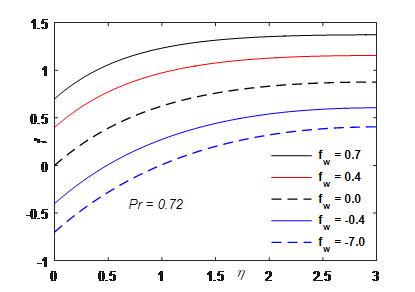Figure 2. Stream function

## 3.png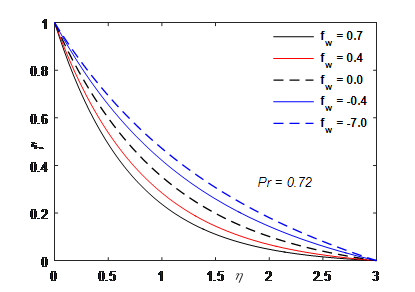Figure 3. Within the boundary layer f'(η) profiles (velocity in the z-direction)

## 4.png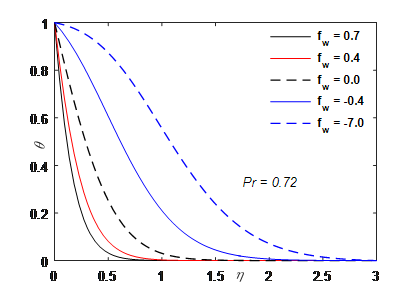Figure 4. Temperature profiles vs fw

## 5.png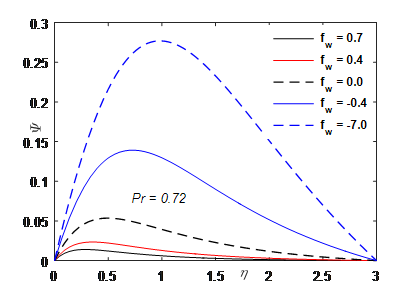Figure 5. Velocity profiles in the z-direction

## 6.png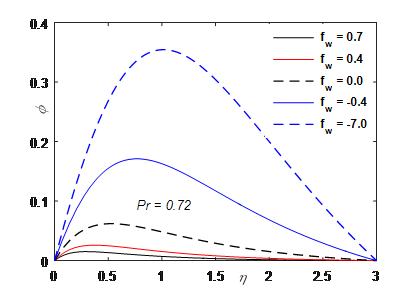Figure 6. Velocity boundary layers in the y-direction

Figures 2 to 6 focus on the temperature and velocity distributions for the outcome of surface mass transfer. The velocity distribution component in z-direction is depicted in Figure 2 where Pr=7.0. There is a lot of influence on the surface mass transfer value. As that of f'(∞)=0, a constant value f(0) is approached f(η) monotonically. The numerical values f(∞) are a measure of entrainment velocity. At the surface, higher entrainment velocity is due to higher suction velocity. Inside the boundary layer, f'(∞) distribution is shown in Figure 3. It can be clearly understood that a more linear shape f'(∞) is obtained by higher values of injection. The distribution of normalized temperature profiles for Pr=7.0 is shown in Figure 4 with varying surface mass parameter. Higher injection values, the thickness of the thermal boundary layer. Alternative behaviors of the thermal boundary layer are observed in the event of higher suction and a decrease of thermal boundary layer exponentially is observed in all specified cases. Maximum values of ψ(η) (velocity component of z-direction of normalized free convection) and φ(η) (velocity component of y-direction in normalized free convection) are located far from the surface on the increasing rate of injection. Figures 5 and 6 clearly, depict the decrease of the velocity boundary layers in an exponential manner. With an increased velocity level, suction is increased. The heat flux at the surface is steep on higher values of injection. This is amalgamated with a temperature reduction at the outer boundary region. The temperature is approximately equal to the ambient temperature as length increases. In case of the rapid increase in atmospheric temperature with height, body temperature rises due to heat transfer and may not be able to achieve the local ambient temperature. When α=0, on a vertically stretched surface, equation 14 describes a zero shear stress $\left( \frac{G{{r}_{x}}}{\operatorname{Re}_{x}^{2}} \right){\psi }'(0)=1$. Zero shear stress or critical value of the boundary layer $\left( \frac{G{{r}_{x}}}{\operatorname{Re}_{x}^{2}} \right)$ is smaller for Prandtl numbers having a lower value than the Prandtl numbers having higher values. For the sample, the value ${{\left( \frac{G{{r}_{x}}}{\operatorname{Re}_{x}^{2}} \right)}_{crit}}$ is 0.1740 at Pr=0.07 whereas its value is 10.79 at Pr=70. For α≠0, velocity is present in all the directions hence, on a rigid surface, there is no rise in induced natural convection in a vertical direction. Alternatively, induced natural convection is slanted in the positive direction of the y-axis, since φ(η) is greater than ψ(η).

5. Conclusions

In this work, The effects of the surface mass transfer on natural convection flow from a vertical stretching surface. Tables are depicted for the missing values of thermal functions and velocity. Predictions on the rate of heat transfer can be performed using this information. Also, the surface friction factor can be estimated. The temperature field and flow field experience a higher influence by surface mass transfer. Higher entrainment velocity is observed at the surface due to suction. Linearity temperature and velocity profiles are higher with injection.

• The thickness of the thermal boundary layer is increased with an increase of injection values and alternatively reduced by surface suction as well as temperature and velocity profiles decay exponentially.
• With an increase of Pr values the result in heat transfer rate increases.
• With minor Prandtl numbers, the thermal boundary layer’s thickness is found to reduce leading to reduced resistance to heat transfer.
Nomenclature
 u,v,w Velocity factors along with the x, y, z directions respectively x,y,z Coordinate directions k Thermal conductivity Gr Grashoff number $\omega$ Conditions at the surface h Heat transfer coefficient Re Reynolds number M Dimensionless velocity along the y-direction N Dimensionless velocity along the x-direction H Dimensionless temperature Nu Nusselt number Pr Prandtl number A Free convection parameter T Temperature $\rho$ Density g Acceleration due to gravity $\alpha$ Angle $\beta$ Coefficient of thermal expansion $\mu$ Viscosity $\eta$ Dimensionless distance $\phi$ Dimensionless velocity $\tau$ Shear stress Cf friction factor $\infty$ Conditions far away from the surface
References

 Surma Devi, C., Takhar, H.S., Nath, G. (1986). Unsteady, three-dimensional, boundary-layer flow due to a stretching surface. International Journal of Heat and Mass Transfer, 29(12): 1996-1999. https://doi.org/10.1016/0017-9310(86)90020-7

 Gupta, P.S., Gupta, A.S. (1977). Heat and mass transfer on a stretching sheet with suction or blowing. The Canadian Journal of Chemical Engineering, 55(6): 744-746. https://doi.org/10.1002/cjce.5450550619

 Chen, C.K., Char, M.I. (1988). Heat transfer of a continuous, stretching surface with suction or blowing. Journal of Mathematical Analysis and Applications, 135(2): 568-580. https://doi.org/10.1016/0022-247X(88)90172-2

 Vajravelu, K. (1994). Convection heat transfer at a stretching sheet with suction or blowing. Journal of Mathematical Analysis and Applications, 188(3): 1002-1011. https://doi.org/10.1006/jmaa.1994.1476

 Bhattacharyya, K. (2011). Effects of heat source/sink on MHD flow and heat transfer over a shrinking sheet with mass suction. Chemical Engineering Research Bulletin, 15(1): 12-17. https://doi.org/10.3329/cerb.v15i1.6524

 Jat, R.N., Chaudhary, S. (2009). MHD flow and heat transfer over a stretching sheet. Applied Mathematical Sciences, 3(26): 1285-1294.

 Fathizadeh, M., Madani, M., Khan, Y., Faraz, N., Yıldırım, A., Tutkun, S. (2013). An effective modification of the homotopy perturbation method for MHD viscous flow over a stretching sheet. Journal of King Saud University-Science, 25(2): 107-113. https://doi.org/10.1016/j.jksus.2011.08.003

 Siri, Z., Ghani, N.A.C., Kasmani, R.M. (2018). Heat transfer over a steady stretching surface in the presence of suction. Boundary Value Problems, 2018(1): 1-16. https://doi.org/10.1186/s13661-018-1019-6

 Daskalakis, J.E. (1992). Free-convection effects in the boundary layer along a vertically stretching flat surface. Canadian Journal of Physics, 70(12): 1253-1260. https://doi.org/10.1139/p92-204

 Ferdows, M., Khaleque, T.S., Bangalee, M.Z.I. (2016). Similarity solution on MHD boundary layer over stretching surface considering heat flux. International Journal of Heat and Technology, 34(3): 521-526. https://doi.org/10.18280/ijht.340325

 Goud, B.S., Shekar, M.N. (2018). Finite element solution of viscous dissipative effects on unsteady MHD flow past a parabolic started vertical plate with mass diffussion and variable temperature. i-Manager's Journal on Mathematics, 7(1): 18-25. https://doi.org/10.26634/jmat.7.1.14028

 Wang, C.Y. (1989). Free convection on a vertical stretching surface. ZAMM. Journal of Applied Mathematics and Mechanics/Zeitschrift für Angewandte Mathematik und Mechanik, 69(11): 418-420. https://doi.org/10.1002/zamm.19890691115

 Bestman, A.R. (1990). Natural convection boundary layer with suction and mass transfer in a porous medium. International Journal of Energy Research, 14(4): 389-396. https://doi.org/10.1002/er.4440140403

 Acharya, M., Singh, L.P., Dash, G.C. (1999). Heat and mass transfer over an accelerating surface with heat source in presence of suction and blowing. International Journal of Engineering Science, 37(2): 189-211. https://doi.org/10.1016/S0020-7225(98)00064-0

 Goud, B.S., Rajashekar, M.N. (2017). Finite element solution on effects of viscous dissipation & diffusion thermo on unsteady mhd flow past an impulsively started inclined oscillating plate with mass diffusion &variable temperature. International Research Journal of Engineering and Technology, 4(2): 471-477.

 Ali, M.E. (1995). On thermal boundary layer on a power-law stretched surface with suction or injection. International Journal of Heat and Fluid Flow, 16(4): 280-290. https://doi.org/10.1016/0142-727X(95)00001-7

 Postelnicu, A., Groşan, T., Pop, I. (2000). Free convection boundary-layer over a vertical permeable flat plate in a porous medium with internal heat generation. International Communications in Heat and Mass Transfer, 27(5): 729-738. https://doi.org/10.1016/S0735-1933(00)00153-6

 Shateyi, S. (2008). Thermal radiation and buoyancy effects on heat and mass transfer over a semi-infinite stretching surface with suction and blowing. Journal of Applied Mathematics, 2008: 414830. https://doi.org/10.1155/2008/414830

 Bhattacharyya, K., Layek, G.C. (2010). Chemically reactive solute distribution in MHD boundary layer flow over a permeable stretching sheet with suction or blowing. Chemical Engineering Communications, 197(12): 1527-1540. https://doi.org/10.1080/00986445.2010.485012

 Shankar Goud, B., Rajashekar, M.N. (2016). Finite element method application of effects on an unsteady MHD convective heat and mass transfer flow in a semi-infinite vertical moving in a porous medium with heat source and suction. IOSR Journal of Mathematics (IOSR-JM), 12(6): 55-64.

 Anil Kumar, M., Dharmendar Reddy, Y., Srinivasa Rao, V., Shankar Goud, B. (2021). Thermal radiation impact on MHD heat transfer natural convective nano fluid flow over an impulsively started vertical plate. Case Studies in Thermal Engineering 24: 100826. https://doi.org/10.1016/j.csite.2020.100826

 Sharma, K., Gupta, S. (2016). Analytical study of MHD boundary layer flow and heat transfer towards a porous exponentially stretching sheet in presence of thermal radiation. International Journal of Advances in Applied Mathematics and Mechanics, 4(1): 1-10.

 Shankar Goud, B. (2020). Heat generation/absorption influence on steady stretched permeable surface on MHD flow of a micropolar fluid through a porous medium in the presence of variable suction/injection. International Journal of Thermofluids, 7-8: 100044. https://doi.org/10.1016/j.ijft.2020.100044

 Naik, H.S., Goud, B.S., Babu, K.R., Murthy, M.R. (2020). Suction/injection effects on free convective fluid flow over a moving vertical porous plate with variable time. Journal of Critical Reviews, 7(18): 1324-1328. http://dx.doi.org/10.31838/jcr.07.18.170

 Goud, B.S., Srilatha, P., Shekar, R. (2019). Effects of mass suction on MHD boundary layer flow and heat transfer over a porous shrinking sheet with heat source/sink. International Journal of Innovative Technology and Exploring Engineering, 8(10): 263-266.

 Tarakaramu, N., Narayana, P.S., Venkateswarlu, B. (2020). Numerical simulation of variable thermal conductivity on 3D flow of nanofluid over a stretching sheet. Nonlinear Engineering, 9(1): 233-243. https://doi.org/10.1515/nleng-2020-0011

 Kumar, M.A, Reddy, Y.D, Goud, B.S, Rao, V.S. (2021). Effects of Soret, Dufour, hall current and rotation on MHD natural convective heat and mass transfer flow past an accelerated vertical plate through a porous medium. International Journal of Thermofluids, 9: 100061. https://doi.org/10.1016/j.ijft.2020.100061

 Srinivasulu, T., Goud, B.S. (2021). Effect of inclined magnetic field on flow, heat and mass transfer of Williamson nanofluid over a stretching sheet. Case Studies in Thermal Engineering, 23: 100819. https://doi.org/10.1016/j.csite.2020.100819

 Goud, B.S., Reddy, Y.D., Rao, V.S., Khan, Z.H. (2020). Thermal radiation and Joule heating effects on a magnetohydrodynamic Casson nanofluid flow in the presence of chemical reaction through a non-linear inclined porous stretching sheet. Journal of Naval Architecture and Marine Engineering, 17(2): 143-164. http://dx.doi.org/10.3329/jname.v17i2.49978

 Gorla, R.S.R., Sidawi, I. (1994). Free convection on a vertical stretching surface with suction and blowing. Applied Scientific Research, 52(3): 247-257. https://doi.org/10.1007/BF00853952

 Ames, W.F. (1965). Nonlinear Partial Differential Equations. New York: Academic Press.